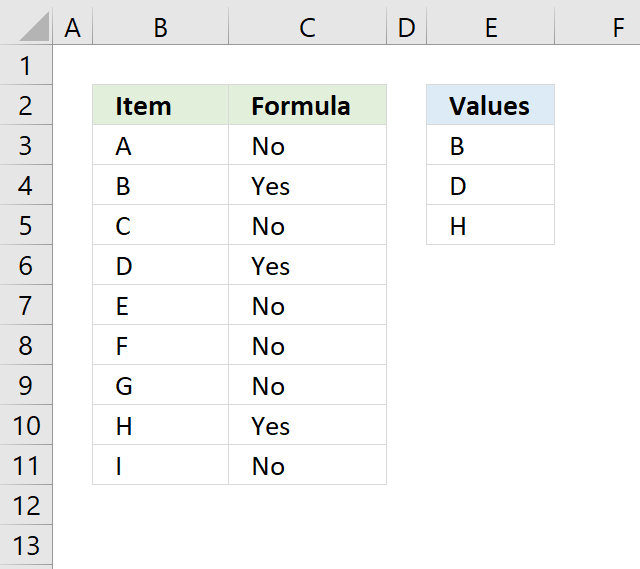Author: Oscar Cronquist Article last updated on October 16, 2018#### Regular formula

Formula in cell C3:

=IF(COUNTIF(\$E\$3:\$E\$5,B3),"Yes","No")

The COUNTIF function counts how many values in E3:E5 match cell B3, it returns 0 (zero) since "A" is not found in E3:A5.

COUNTIF(\$E\$3:\$E\$5,B3)

becomes

COUNTIF({"B";"D";"H"},"A")

and returns 0 (zero).

The IF function then returns "YES" if the logical expression is 1 or more, "No" if the logical expression returns 0 (zero).

IF(COUNTIF(\$E\$3:\$E\$5,B3),"Yes","No")

becomes

IF(0,"Yes","No") and returns "No" in cell C3.

#### Array formula

I recommend using the regular formula above, this array formula checks if cell B3 is equal to any of the values in E3:E5, the IF function returns Yes if one of the values is a match and No if none of the values match.

=IF(OR(B3=\$E\$3:\$E\$5),"Yes","No")

To enter an array formula, type the formula in a cell and then press and hold CTRL + SHIFT simultaneously, now press Enter once. Release all keys.

The formula bar now shows the formula enclosed with curly brackets telling you that you entered the formula successfully. Don't enter the curly brackets yourself.

The equal sign compares the value in cell B3 with each value in cell range E3:E5.

=IF(OR("A"={"B";"D";"H"}),"Yes","No")

If the cell matches a value in E3:E5 it returns TRUE, if not FALSE. In this case, all logical expressions return FALSE.

=IF(OR({FALSE;FALSE;FALSE}),"Yes","No")

The OR function returns FALSE since all arguments are FALSE.

=IF(FALSE,"Yes","No")

The IF function then returns No in cell C3.

### Alternative regular formula

The INDEX function changes the above formula into a regular formula.

=IF(OR(INDEX(B3=\$E\$3:\$E\$5,)),"Yes","No")

The INDEX and SUMPRODUCT functions have this characteristic, you just have to know when and where to apply them.

### Related post

Use IF + COUNTIF to perform numerous conditions

The COUNTIF function allows you to construct a small IF formula that carries out plenty of logical expressions. Combining the IF […]